8.4.1 Steps 1 and 2: Search and Insert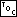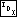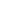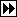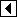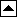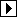The first step is a lot like the unthreaded AVL version in <Step 1: Search AVL tree for insertion point 148>. There is an unfortunate special case for an empty tree, because a null pointer for tavl_root indicates an empty tree but in a nonempty tree we must seek a thread link. After we're done, p, not q as before, is the node below which a new node should be inserted, because the test for stepping outside the binary tree now comes before advancing p.

```302. <Step 1: Search TAVL tree for insertion point 302> =
z = (struct tavl_node *) &tree->tavl_root;
y = tree->tavl_root;
if (y != NULL)   {
for (q = z, p = y; ; q = p, p = p->tavl_link[dir])       {
int cmp = tree->tavl_compare (item, p->tavl_data, tree->tavl_param);
if (cmp == 0)
return &p->tavl_data;

if (p->tavl_balance != 0)
z = q, y = p, k = 0;
da[k++] = dir = cmp > 0;

if (p->tavl_tag[dir] == TAVL_THREAD)
break;
}
} else   {
p = z;
dir = 0;
}
```

This code is included in 301.

The insertion adds to the TBST code by setting the balance factor of the new node and handling the first insertion into an empty tree as a special case:

```303. <Step 2: Insert TAVL node 303> =
<Step 2: Insert TBST node; tbst => tavl 256>
n->tavl_balance = 0;
if (tree->tavl_root == n)
return &n->tavl_data;
```

This code is included in 301.